##By Brooke Wert

Recognize a line of symmetry for a two-dimensional figure as a line across the figure such that the figure can be folded along the line into matching parts.# Symmetry and Symmetrical Figures

##By Magicpathashala

What is Symmetry# Lines, Rays, Line Segments, Points, Angles, Union & Intersection - Geometry Basic Introduction

##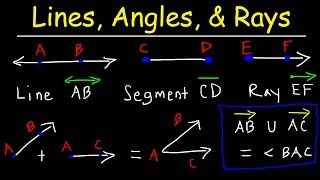By The Organic Chemistry Tutor

basic introduction into lines, rays, line segments, points, and angles. It also explains the difference between the union and intersection symbols and contains a few practice problems associated with it.# [4.G.3-1.0] Lines of Symmetry - Common Core Standard

##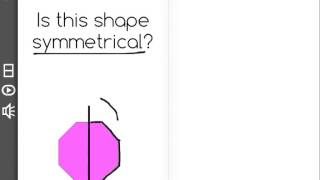By Freckle education

Recognize a line of symmetry for a two-dimensional figure as a line across the figure such that the figure can be folded along the line into matching parts.# Lines of Symmetry

##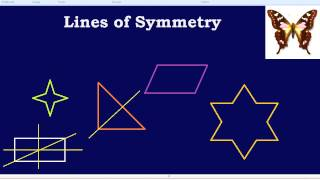By Bespoke education

How to find lines of symmetry.# Math Antics - Points, Lines, & Planes

##By mathanatics

Draw points, lines, line segments, rays, angles (right, acute, obtuse), and perpendicular and parallel lines. Identify these in two-dimensional figures.# Acute Triangle - YourTeacher.com - Geometry Help

##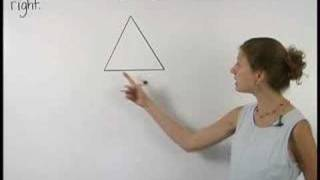By yourteachermathhelp

In this lesson students learn to classify triangles as acute (all angles acute) right (one angle right) or obtuse (one angle obtuse). Students also learn that the sum of the measures of the angles of a triangle is 180 degrees and students are asked to use this formula to find the measures of missing angles in given triangles.# Introduction to Symmetry

##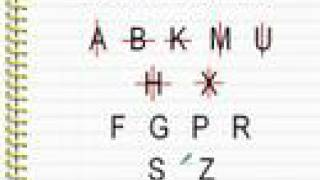By mathwithlarry

Introductory lesson concerning symmetry. Instructor Larry explains the general idea of symmetry and gives examples with letters in this clip. The instructor uses computer software for demonstration.# Point Line and Line Segment

##By TopperLearning

Point Line and Line Segment##By Khan Academy

Let's practice categorizing triangles in this example exercise.# Identifying parallel and perpendicular lines | Geometry | Khan Academy

##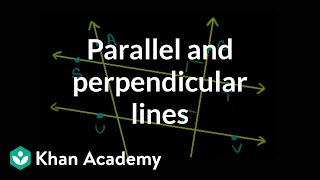By KhanAcademy

identify parallel and perpendicular lines# Axis of symmetry

##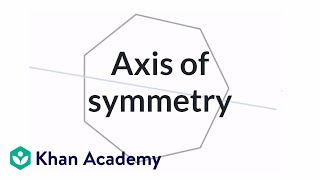By Khan Academy

Sal introduces the concept of an axis of symmetry.167# Flipped Classroom Lines and Angles

##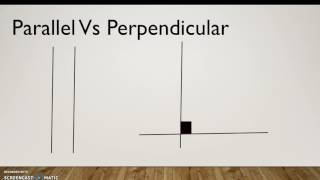By Megan Priole

Draw points, lines, line segments, rays, angles (right, acute, obtuse), and perpendicular and parallel lines.# Point Line Plane

##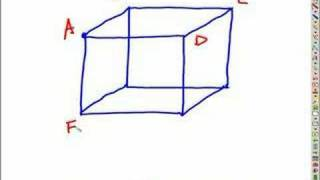By Pete Anderson

The instructor uses an electronic sketchpad to explain and demonstrates the fundamental concepts (undefined terms)of geometry: point line plane.# Recognizing parallel and perpendicular lines

##By Khan Academy

Let's now apply what we learned and do an exercise together in which we identify parallel and perpendicular lines.This is great practice.# Recognizing parallel and perpendicular lines

##By Khan Academy

Do the two lines intersect or stay apart? If they intersect, do they do so thereby creating a 90 degree angle? These are the kinds of questions we ask ourselves when we learn about parallel and perpendicular lines.# Recognizing angles

##By Khan Academy

Let's do a few example exercises in which you recognize and identify the type of given angle. This is a lot of fun!# Parallel and Perpendicular Lines, Transversals, Alternate Interior Angles, Alternate Exterior Angles

##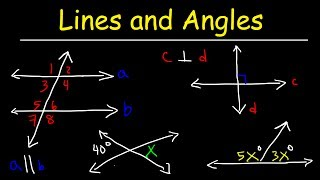By The Organic Chemistry Tutor

parallel and perpendicular lines in addition to transversals and all the angles that are formed by it such as alternate interior and exterior angles.# Grade 5 Math - Classifying Plane (2-D) Shapes 7

##By Lumos Learning

Using the Lumos Study Programs, parents and educators can reinforce the classroom learning experience for children and help them succeed at school and on the standardized tests. Lumos books, dvd, eLearning and tutoring are used by leading schools, libraries and thousands of parents to supplement classroom learning and improve student achievement in the standardized tests.##By Khan Academy